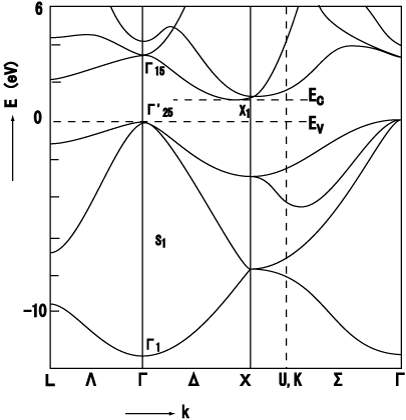# Reading Band structure plotsI know that there are similar questions already answered here on Physics StackExchange but the following things are still unclear to me:

1. Is it correct that an electron inside a crystal with a band structure shown above can have any energy inside the band? What is this energy (neglecting such things as external magnetic fields etc.)?

2. How do I have to read these types of plots, i.e. where are the gaps? Or stated differently: How can I know from the picture which are the filled bands and which are the gaps? Is the region below the first line with an energy between -7 and -14 eV a band or a gap?

3. How comes that there are bands but if I consider only one atom this has a parabolic energy dispersion with only one allowed energy for every k-vector. I have seen the calculation for two atoms and how there appears a gap. But there the electrons still can have only two energies per k-vector and I can't really make the jump to the continous bands. Is this under the (justified) assumption that there are infinitely many atoms? Unfortunately, every textbook makes this jump directly without ever explaining it rigorously, i.e. with a calculation (altough it kind of makes sense).

• 3) For one atom, the electron energies are discrete. Without translation symmetry, there is no k-vector. Same for a molecule. – user137289 Mar 3 '18 at 18:30
• @Pieter: Does this mean that the electron can have every energy inside the band? How do I know from the plot whether I am in a band or in a gap? – Quasar Mar 4 '18 at 17:26
• The lines are dispersion relations in different high-symmetry directions. Yes, for each energy in a band there are allowed states. The only gap here (no states) is between 0 eV and 1 eV. It seems to me you should read your textbook. – user137289 Mar 4 '18 at 22:45
• @Pieter: The issue is the following: If I have a band it has an upper and a lower boundary. How do I know from the plot which line is corresponds to which band and which other band is its corresponding upper or lower counterpart for this band? – Quasar Mar 5 '18 at 7:58
• It is not as simple as in chemistry with its bonding and antibonding molecular orbitals (which also is an approximation of real quantum-chemical calculations). It is easiest för $k$ at zone boundaries, where one has standing waves. For example at the $L$ point in this figure, where there is a gap around -8 eV. The lower energy value has the electron density at the atoms ("bonding state"), the higher energy value has the electron density between the atoms ("antibonding state" in molecular-orbital jargon). Books by Walter Harrison are good for tight-binding theory and connections to chemistry. – user137289 Mar 5 '18 at 9:25

## 1 Answer

Yes, this plot is a little tricky to gain intuition on right away. I am still working on it myself. Here's what I understand about this.

1) Each line is an eigenmode, which for that particular material is the allowable fundamental modes for given wavenumbers

2) The x axis corresponds to the physical space of the unit cell. This labeling depends on the how you setup your fundamental unit. In periodic structures, you are able to describe most of the physics by simplifying it's features to the smallest unit that describes most of the properties.

3) The y axis gives you the amount of energy

Let's walk through an example :

1) Start at one tick on the x axis (gamma for starters) and go up along the y-axis.

2) Once you run into a line that means you ran into the first eigenmode

3) If you continue up then you run into the second line, which is the second eigenmode

4) Each jump between lines you need to make is a gap

5) For the lower energy states (like the one in these pictures) there are big distinctions between each allowable energy state. When you get up to higher energies than there are not very large gaps between eigenmodes, which makes it more into a continuum of allowable energies.

6) Things get 'upgraded' to a bandgap when these gaps extend across a broad range of x values.

Hope that helps!

• The $x$ axis is not the physical space of the unit cell. It is the space of reciprocal lattice vectors. A gap is an energy for which there is no state. In this case, which is an indirect gap, You don't find gaps by going up the $\Gamma$ point line. In the example shown the gap is between the dashed horizontal lines. The post needs cleaning up, which I'll help with if I have time. – garyp May 11 '20 at 16:21